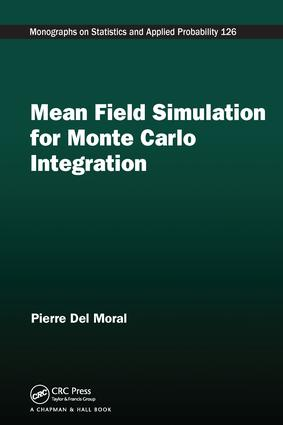Mean Field Simulation for Monte Carlo Integration

1st Edition

Chapman and Hall/CRC

626 pages | 9 B/W Illus.

Paperback: 9781138198739
pub: 2016-10-26
\$64.95
x
Hardback: 9781466504059
pub: 2013-05-20
\$165.00
x
eBook (VitalSource) : 9780429086960
pub: 2013-05-20
from \$31.48

FREE Standard Shipping!

Description

In the last three decades, there has been a dramatic increase in the use of interacting particle methods as a powerful tool in real-world applications of Monte Carlo simulation in computational physics, population biology, computer sciences, and statistical machine learning. Ideally suited to parallel and distributed computation, these advanced particle algorithms include nonlinear interacting jump diffusions; quantum, diffusion, and resampled Monte Carlo methods; Feynman-Kac particle models; genetic and evolutionary algorithms; sequential Monte Carlo methods; adaptive and interacting Markov chain Monte Carlo models; bootstrapping methods; ensemble Kalman filters; and interacting particle filters.

Mean Field Simulation for Monte Carlo Integration presents the first comprehensive and modern mathematical treatment of mean field particle simulation models and interdisciplinary research topics, including interacting jumps and McKean-Vlasov processes, sequential Monte Carlo methodologies, genetic particle algorithms, genealogical tree-based algorithms, and quantum and diffusion Monte Carlo methods.

Along with covering refined convergence analysis on nonlinear Markov chain models, the author discusses applications related to parameter estimation in hidden Markov chain models, stochastic optimization, nonlinear filtering and multiple target tracking, stochastic optimization, calibration and uncertainty propagations in numerical codes, rare event simulation, financial mathematics, and free energy and quasi-invariant measures arising in computational physics and population biology.

This book shows how mean field particle simulation has revolutionized the field of Monte Carlo integration and stochastic algorithms. It will help theoretical probability researchers, applied statisticians, biologists, statistical physicists, and computer scientists work better across their own disciplinary boundaries.

Reviews

"…I found this to be an enjoyable read. Many illustrative examples reveal intriguing paradoxes in statistical theories, some of them are well-known and complemented with a broad informative discussion and others are less obvious."

—Journal of the American Statistical Association

Monte Carlo and Mean Field Models

Linear evolution equations

Nonlinear McKean evolutions

Time discretization schemes

Illustrative examples

Mean field particle methods

Theory and Applications

A stochastic perturbation analysis

Feynman-Kac particle models

Extended Feynman-Kac models

Nonlinear intensity measure equations

Statistical machine learning models

Risk analysis and rare event simulation

Feynman-Kac Models

Discrete Time Feynman-Kac Models

A brief treatise on evolution operators

Feynman-Kac models

Some illustrations

Historical processes

Feynman-Kac sensitivity measures

Four Equivalent Particle Interpretations

Spatial branching models

Sequential Monte Carlo methodology

Interacting Markov chain Monte Carlo algorithms

Mean field interacting particle models

Continuous Time Feynman-Kac Models

Some operator aspects of Markov processes

Feynman-Kac models

Continuous time McKean models

Mean field particle models

Nonlinear Evolutions of Intensity Measures

Intensity of spatial branching processes

Nonlinear equations of positive measures

Multiple-object nonlinear filtering equations

Association tree-based measures

Application Domains

Particle Absorption Models

Particle motions in absorbing medium

Mean field particle models

Some illustrations

Absorption models in random environments

Particle Feynman-Kac models

Doob h-processes

Signal Processing and Control Systems

Nonlinear filtering problems

Linear Gaussian models

Interacting Kalman filters

Quenched and annealed filtering models

Particle quenched and annealed models

Parameter estimation in hidden Markov models

Optimal stopping problems

Theoretical Aspects

Mean Field Feynman-Kac Models

Feynman-Kac models

McKean-Markov chain models

Perfect sampling models

Interacting particle systems

Some convergence estimates

Continuous time models

A General Class of Mean Field Models

Description of the models

Some weak regularity properties

Some illustrative examples

A stochastic coupling technique

Fluctuation analysis

Empirical Processes

Description of the models

Nonasymptotic theorems

A reminder on Orlicz’s norms

Finite marginal inequalities

Maximal inequalities

Cramér-Chernov inequalities

Perturbation analysis

Interacting processes

Feynman-Kac Semigroups

Description of the models

Stability properties

Semigroups of nonlinear Markov chain models

Backward Markovian semigroups

Intensity Measure Semigroups

Spatial branching models

Measure-valued nonlinear equations

Weak Lipschitz properties of semigroups

Stability properties of PHD models

Particle Density Profiles

Stochastic perturbation analysis

First order expansions

Some nonasymptotic theorems

Fluctuation analysis

Concentration inequalities

A general class of mean field particle models

Particle branching intensity measures

Positive measure particle equations

Genealogical Tree Models

Some equivalence principles

Some nonasymptotic theorems

Ancestral tree occupation measures

Central limit theorems

Concentration inequalities

Particle Normalizing Constants

Unnormalized particle measures

Some key decompositions

Fluctuation theorems

A nonasymptotic variance theorem

Lp-mean error estimates

Concentration analysis

Backward Particle Markov Models

Description of the models

Conditioning principles

Integral transport properties

A stochastic perturbation analysis

Orlicz norm and Lm-mean error estimates

Some nonasymptotic variance estimates

Fluctuation analysis

Concentration inequalities

Bibliography

Index

Pierre Del Moral is a professor in the School of Mathematics and Statistics at the University of New South Wales in Sydney, Australia.